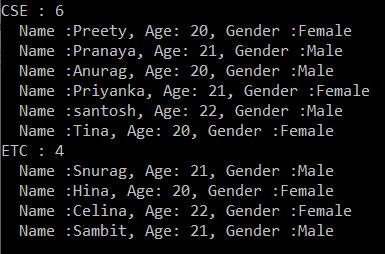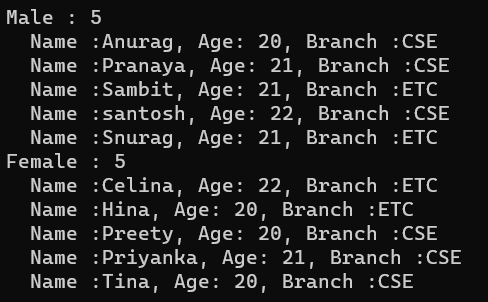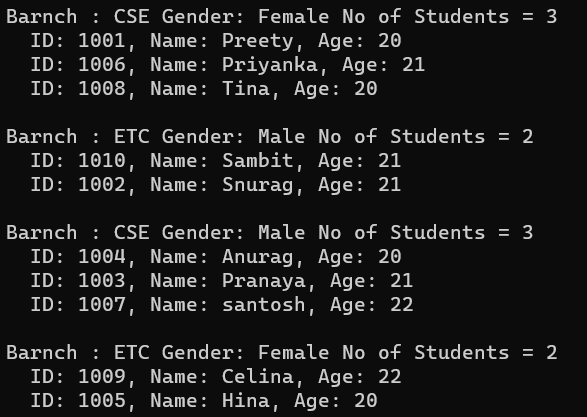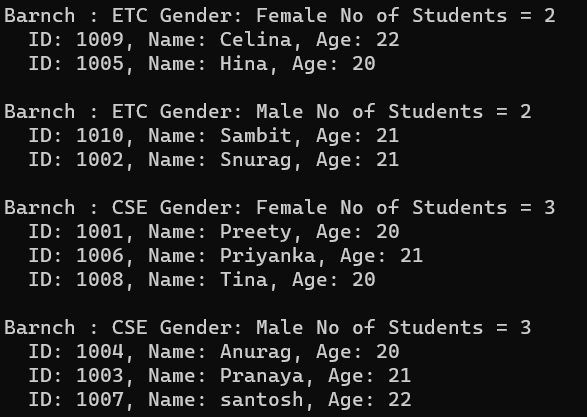# LINQ ToLookup Method in C#

## LINQ ToLookup Method in C# with Examples

In this article, I will discuss the LINQ ToLookup Method in C# with Examples. Please read our previous article, discussing the LINQ GroupBy Method with Multiple Keys in C# with Examples. As part of this article, we will discuss the following pointers.

1. What is the LINQ ToLookup Method in C#?
2. Examples of using the ToLookup Method in C# using both Method and Query Syntax.
3. How do you use the ToLookup method with the OrderBy method?
4. How to use the ToLookup method in C# with Multiple Keys?
5. What is the difference between ToLookup and GroupBy Methods in Linq?
6. When to Use LINQ ToLookup Method in C#?
##### What is the LINQ ToLookup Method in C#?

The LINQ ToLookup method in C# creates a Lookup<TKey, TElement>, a special data structure similar to a dictionary. A Lookup resembles a Dictionary in that it maps keys to collections of values, but with a few differences:

• Allowance for Multiple Values per Key: Each key in a Lookup can be associated with a sequence of values rather than a single value, as in a dictionary.
• Immutable: Once a Lookup is created, it cannot be modified; it’s read-only.

The LINQ ToLookup Method in C# exactly does the same thing as the GroupBy Method. The only difference between these two methods is the GroupBy method uses Deferred Execution, whereas the ToLookup method uses Immediate Execution. Before proceeding further, let us first understand what Deferred Execution and Immediate Execution are in LINQ.

##### What is LINQ Deferred Execution?

In the case of Deferred Execution, the LINQ Query is not executed at the point of its declaration. That means it doesn’t execute by itself when we write a LINQ query. It executes only when we access the query results. So, here, the execution of the query is deferred until the query variable is iterated over using a for-each loop. For example, Select, SelectMany, Where, Take, Skip, etc., belong to the Deferred or Lazy Operators Category.

##### What is LINQ Immediate Execution?

In the case of Immediate Execution, the LINQ query is executed at the point of its declaration. So, it forces the query to execute and gets the result immediately, for example. Count, Average, Min, Max, First, Last, ToArray, ToList, etc., belongs to the Immediate or Greedy Operators category. Please read the following article to understand what Deferred and Immediate Execution are in Linq Queries in detail with examples.

Deferred Execution VS Immediate Execution in C#.

##### LINQ ToLookup Method in C# with Examples

Let us understand how to use the LINQ ToLookup Method in C# using both Method and Query Syntax with Examples. We will use the following Student class to understand the ToLookup Method. So, create a class file named Student.cs and copy and paste the following code. The following Student class contains five properties: ID, Name, Gender, Branch, and Age. This class also has one method called GetStudents(), which returns a list of all students and will be our data source.

```using System.Collections.Generic;
namespace ToLookupDemo
{
public class Student
{
public int ID { get; set; }
public string Name { get; set; }
public string Gender { get; set; }
public string Branch { get; set; }
public int Age { get; set; }

public static List<Student> GetStudents()
{
return new List<Student>()
{
new Student { ID = 1001, Name = "Preety", Gender = "Female", Branch = "CSE", Age = 20 },
new Student { ID = 1002, Name = "Snurag", Gender = "Male", Branch = "ETC", Age = 21  },
new Student { ID = 1003, Name = "Pranaya", Gender = "Male", Branch = "CSE", Age = 21  },
new Student { ID = 1004, Name = "Anurag", Gender = "Male", Branch = "CSE", Age = 20  },
new Student { ID = 1005, Name = "Hina", Gender = "Female", Branch = "ETC", Age = 20 },
new Student { ID = 1006, Name = "Priyanka", Gender = "Female", Branch = "CSE", Age = 21 },
new Student { ID = 1007, Name = "santosh", Gender = "Male", Branch = "CSE", Age = 22  },
new Student { ID = 1008, Name = "Tina", Gender = "Female", Branch = "CSE", Age = 20  },
new Student { ID = 1009, Name = "Celina", Gender = "Female", Branch = "ETC", Age = 22 },
new Student { ID = 1010, Name = "Sambit", Gender = "Male",Branch = "ETC", Age = 21 }
};
}
}
}```
##### Grouping the Students Based on Branch using LINQ ToLookup Method in C#

Now, our requirement is to group the students based on their Branch. For a better understanding, please look at the following example, which exactly does the same using the ToLookup Method with both Method and Query Syntax. The following example organizes the students into groups based on their branch (i.e., the branch will act as the key). It also means that students with the same branch will be stored in the same group, where each group has a key and the corresponding student collection. Here, the key will be the Branch, and the collection will be the student belonging to that particular branch. The following example code is self-explained, so please go through the comment lines.

```using System;
using System.Linq;
namespace ToLookupDemo
{
class Program
{
static void Main(string[] args)
{
//Grouping the Students Based on Branch using ToLookup Method

//Using Method Syntax
var GroupByMS = Student.GetStudents().ToLookup(s => s.Branch);

//Using Query Syntax
var GroupByQS = (from std in Student.GetStudents()
select std).ToLookup(x => x.Branch);

//It will iterate through each group
foreach (var group in GroupByMS)
{
Console.WriteLine(group.Key + " : " + group.Count());

//Iterate through each student of a group
foreach (var student in group)
{
Console.WriteLine("  Name :" + student.Name + ", Age: " + student.Age + ", Gender :" + student.Gender);
}
}

}
}
}```
###### Output:Note: Each group has a key, and you can access the value of the key by using the key property. Along the same line, you can also use the count property to check how many elements are in that group. Again, you can access all the group elements shown in the above example using a for each loop.

##### Key Features of LINQ ToLookup  Method in C#:
• Grouping Elements: ToLookup is typically used for grouping elements into a collection where keys can have multiple associated values.
• Deferred Execution: The method uses deferred execution for grouping, but the resulting Lookup is immutable and executes immediately.
• Handling of Non-Existent Keys: When querying a non-existent key, ToLookup returns an empty sequence instead of throwing an exception (unlike a dictionary).
• Applicability: Useful in scenarios where data needs to be categorized and accessed by keys, especially when keys can be associated with multiple values.
##### Grouping Students by Gender in Descending Order, Names in Ascending Order in Each Group

Let us see an example to Understand the LINQ ToLookup Method with the following Requirements.

1. First, Grouping the Students by Gender.
2. Then, sort the Groups in Descending Order, i.e., soring the gender in Descending Order.
3. Finally, Sort the Student Names in each group in Ascending Order.

In the following example, we group the students by Gender using the LINQ ToLookup Method. But here, we first sort the data by Gender in Descending Order and then sort the student in each group by their name in Ascending Order. The following example code is self-explained, so please go through the comment lines.

```using System;
using System.Linq;

namespace ToLookupDemo
{
class Program
{
static void Main(string[] args)
{
//Using Method Syntax
//First Group the Data by Gender using ToLookup Method
var GroupByMS = Student.GetStudents().ToLookup(s => s.Gender)
//Then Sorting the data based on key in Descending Order
.OrderByDescending(c => c.Key)
.Select(std => new
{
Key = std.Key,
//Sorting the Students in Each Group based on Name in Ascending order
Students = std.OrderBy(x => x.Name)
});

//Using Query Syntax
var GroupByQS = (from std in Student.GetStudents()
select std).ToLookup(x => x.Gender)
//Then Sorting the data based on key in Descending Order
.OrderByDescending(c => c.Key)
.Select(std => new
{
Key = std.Key,
//Sorting the Students in Each Group based on Name in Ascending order
Students = std.OrderBy(x => x.Name)
});

//It will iterate through each groups
foreach (var group in GroupByQS)
{
Console.WriteLine(group.Key + " : " + group.Students.Count());

//Iterate through each student of a group
foreach (var student in group.Students)
{
Console.WriteLine("  Name :" + student.Name + ", Age: " + student.Age + ", Branch :" + student.Branch);
}
}

}
}
}```
###### Output:We have projected the result to an anonymous type if you observe the above example. If you want, instead of an anonymous type, you can also create a new type with the required properties, and then you can project the result to that new type. Let us understand this with an example. First, create a class file named StudentGroup.cs and copy and paste the following code.

```using System.Collections.Generic;
namespace ToLookupDemo
{
public class StudentGroup
{
public string Key { get; set; }
public List<Student> Students { get; set; }
}
}```

With the above StudentGroup class in place, now modify the Main method of the Program class as follows. Here, you can see we are projecting the result into the newly created StudentGroup type instead of the anonymous type.

```using System;
using System.Linq;
namespace ToLookupDemo
{
class Program
{
static void Main(string[] args)
{
//Using Method Syntax
//First Group the Data by Gender using ToLookup Method
var GroupByMS = Student.GetStudents().ToLookup(s => s.Gender)
//Then Sorting the data based on key in Descending Order
.OrderByDescending(c => c.Key)
.Select(std => new StudentGroup
{
Key = std.Key,
//Sorting the Students in Each Group based on Name in Ascending order
Students = std.OrderBy(x => x.Name).ToList()
});

//Using Query Syntax
var GroupByQS = (from std in Student.GetStudents()
select std).ToLookup(x => x.Gender)
//Then Sorting the data based on key in Descending Order
.OrderByDescending(c => c.Key)
.Select(std => new StudentGroup
{
Key = std.Key,
//Sorting the Students in Each Group based on Name in Ascending order
Students = std.OrderBy(x => x.Name).ToList()
});

//It will iterate through each groups
foreach (var group in GroupByQS)
{
Console.WriteLine(group.Key + " : " + group.Students.Count());

//Iterate through each student of a group
foreach (var student in group.Students)
{
Console.WriteLine("  Name :" + student.Name + ", Age: " + student.Age + ", Branch :" + student.Branch);
}
}

}
}
}```

Run the application, and you will get the same output shown in the image below.##### Using LINQ ToLookup Method with Multiple Keys in C#:

Let us understand how to use the LINQ ToLookup Method in C# with Multiple Keys. Our requirement is to group the students first based on Branch and then by Gender. That means, first, we need to group the students by Branch, and then we need to group the students by Gender. For a better understanding, please look at the following example, which exactly does the same thing. The following example code is self-explained, so please go through the comment lines.

```using System;
using System.Linq;
namespace ToLookupDemo
{
class Program
{
static void Main(string[] args)
{
//Grouping Students by Branch and Gender using ToLookup Method
var GroupByMultipleKeysMS = Student.GetStudents()
.ToLookup(x => new { x.Branch, x.Gender })
.Select(g => new
{
Branch = g.Key.Branch,
Gender = g.Key.Gender,
Students = g.OrderBy(x => x.Name)
}); ;

//It will iterate through each group
foreach (var group in GroupByMultipleKeysMS)
{
Console.WriteLine(\$"Barnch : {group.Branch} Gender: {group.Gender} No of Students = {group.Students.Count()}");
//It will iterate through each item of a group
foreach (var student in group.Students)
{
Console.WriteLine(\$"  ID: {student.ID}, Name: {student.Name}, Age: {student.Age} ");
}
Console.WriteLine();
}

}
}
}```
###### Output:##### Grouping Students Based on the Branch and Gender along with OrderBy Method

Our requirement is to group the students first by Branch and then by Gender. First, we need to group the students by Branch in Descending Order, and then we need to group the students by Gender in Ascending order. Finally, the students in each group need to be sorted by their names in Ascending Order. For a better understanding, please look at the following example, which exactly does the same thing. The following example code is self-explained, so please go through the comment lines.

```using System;
using System.Linq;
namespace ToLookupDemo
{
class Program
{
static void Main(string[] args)
{
//Using Method Syntax
var GroupByMultipleKeysMS = Student.GetStudents()
//Group the Students first by Branch and then by Gender using ToLookup
.ToLookup(x => new { x.Branch, x.Gender })
//Sort Each Group in Descending Order Based on Branch
.OrderByDescending(g => g.Key.Branch)
//Then Sort Each Branch Group in Ascending Order Based on Gender
.ThenBy(g => g.Key.Gender)
//Project the Result to an Annonymous Type
.Select(g => new
{
Branch = g.Key.Branch,
Gender = g.Key.Gender,
//Sort the Students of Each group by Name in Ascending Order
Students = g.OrderBy(x => x.Name)
});

//It will iterate through each group
foreach (var group in GroupByMultipleKeysMS)
{
Console.WriteLine(\$"Barnch : {group.Branch} Gender: {group.Gender} No of Students = {group.Students.Count()}");
//It will iterate through each item of a group
foreach (var student in group.Students)
{
Console.WriteLine(\$"  ID: {student.ID}, Name: {student.Name}, Age: {student.Age} ");
}
Console.WriteLine();
}
}
}
}```
###### Output:We have projected the result to an anonymous type in the above example. You can also create a new type with the required properties and then project the result to that new type. Let us understand this with an example. First, create a class file named StudentGroupByBranchAndGender.cs and copy and paste the following code.

```using System.Collections.Generic;
namespace ToLookupDemo
{
public class StudentGroupByBranchAndGender
{
public string Branch { get; set; }
public string Gender { get; set; }
public List<Student> Students { get; set; }
}
}```

With the above changes in place, now modify the Main method of the Program class as follows. Here, we are projecting the result into the newly created StudentGroupByBranchAndGender type instead of the anonymous one. The following example code is self-explained, so please go through the comment lines for a better understanding.

```using System;
using System.Linq;
namespace ToLookupDemo
{
class Program
{
static void Main(string[] args)
{
//Using Method Syntax
var GroupByMultipleKeysMS = Student.GetStudents()
//Group the Students first by Branch and then by Gender using ToLookup
.ToLookup(x => new { x.Branch, x.Gender })
//Sort Each Group in Descending Order Based on Branch
.OrderByDescending(g => g.Key.Branch)
//Then Sort Each Branch Group in Ascending Order Based on Gender
.ThenBy(g => g.Key.Gender)
//Project the Result to an Annonymous Type
.Select(g => new StudentGroupByBranchAndGender
{
Branch = g.Key.Branch,
Gender = g.Key.Gender,
//Sort the Students of Each group by Name in Ascending Order
Students = g.OrderBy(x => x.Name).ToList()
});

//It will iterate through each group
foreach (var group in GroupByMultipleKeysMS)
{
Console.WriteLine(\$"Barnch : {group.Branch} Gender: {group.Gender} No of Students = {group.Students.Count()}");
//It will iterate through each item of a group
foreach (var student in group.Students)
{
Console.WriteLine(\$"  ID: {student.ID}, Name: {student.Name}, Age: {student.Age} ");
}
Console.WriteLine();
}
}
}
}```

When you run the above code, you will get the same output as the previous example, as shown in the below image.##### When to Use LINQ ToLookup Method in C#?

The LINQ ToLookup method in C# is ideal for organizing elements into a collection based on a key, and each key can be associated with multiple values. It creates a Lookup<TKey, TElement>, similar to a dictionary but more suited for certain scenarios. Here are some typical use cases:

• Grouping Data: When you have a collection of items you want to group by a particular property. For instance, grouping a list of employees by department or a list of transactions by date.
• Data Categorization: ToLookup categorizes data for easy access and manipulation. For example, categorizing products by their categories or customers by their regions.
• Efficient Data Retrieval: If you frequently need to access elements by a key and each key can have multiple associated values, a Lookup provides an efficient way to retrieve these grouped elements.
• Preventing Key Errors: Unlike a dictionary, accessing a non-existent key in a Lookup returns an empty sequence instead of throwing an exception. This makes it useful in scenarios where keys might not be present.
• Data Transformation and Analysis: In scenarios where you need to transform data into a grouped format for analysis or reporting, ToLookup can be a handy method to simplify this process.
• Joining Operations: ToLookup can be used to optimize join operations, especially in scenarios where one side of the join has multiple matching elements on the other side.
• Creating a Read-Only, Grouped View of Data: Since a Lookup is immutable, it’s a good choice when you need a read-only, grouped view of your data.

In the next article, I will discuss the LINQ Joins in C# with Examples. In this article, I explain the LINQ ToLookup Method in C# with Examples. I hope you understand the need and use of the Linq ToLookup Method in C# with Examples.